# Contrasts of Meaning and Purpose

 < Day Day Up >

Let us write fOLS(x) as a model for the data based on the OLS criterion, and similarly f*(x) as a, say, ceiling frontier model. Then we have two representations of the data values, yj. Namely,

 (5)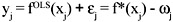We note first that the two model values can and will likely be different. Which is valid or perhaps most valid? We can apply the test of normality to the OLS residuals and the NLOB criterion to the frontier residuals as an obvious first step that may support a choice. But what if both tests are acceptable? Then we suggest relying on context. Namely, we ask whether the value yj can be regarded as in the nature of an attempt at getting high values. If so, then the ceiling model would appear more appropriate. By contrast, if these data values are thought to be merely random deviations from a mean response then the OLS model should be preferred. A familiar example is that of a set of test scores for a student examination. Either case or even mixtures of these cases might apply. If the exam were in a required course, for which an average grade is most desirable for the majority of the students, then the OLS model is compelling. On the other hand, if the test is a college entrance or professional qualification exam, then a higher grade is likely to be better for most students. In that case, the frontier model would likely be best.

Such difficult-to-call cases might be especially expected to occur with large sample sizes. As an illustration, we consider an example in Madansky (1988). There a large data set of 100 observations was simulated according to a gamma distribution anchored at zero. Then various well-known tests of normality were applied to see whether they could correctly reject the normal distribution hypothesis. Surprisingly, several of the tests did not reject the normal hypothesis. The gamma distribution chosen appeared to be well modeled by a normal density according to several tests.

This example leads to several observations in connection with the representation (5). First let us denote by a the average of the frontier residuals, ωj. Next we write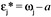. Then. Substitution into (5) yields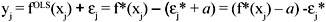or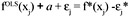One is tempted to suggest in such a case that a suitable frontier model may then be obtained from the OLS model by translating it upwards by the amount a. However, this would require the two types of epsilon residuals to be equal and opposite in sign. That this is not generally true is most easily seen from Figure 2. Namely, if the OLS model were translated upward it would apparently intersect the frontier model. Thus even if the frontier residuals are normally distributed, the frontier model may differ from the OLS model adjusted for the mean frontier residuals. In this case we may even assume that the frontier model was fitted by an OLS criterion with the constraint of passing through the origin. As the fitting criterion is changed and more complex constraints are present, it is reasonable to expect differences between the forms of models obtained—despite the possibility of normally distributed residuals for both models.

Moreover, the foregoing points suggest the possibility of obtaining somewhat misleading regression analyses on comparative performance data analysis. Let us consider the context of explaining the performances of firms according to a single independent variable, x. We might think of x as some measure such as size in dollar valuation of assets, say, along with a dependent variable, y, as some acceptable measure of performance. For simplicity, we assume that the performance variable is not directly proportional to size. The analyst may well obtain an acceptable OLS model for such data. Perhaps the fitted model suggests that y = a0 + b0x explains the firm performance data very well. However, as an OLS regression model, this result can be interpreted in an average or typical sense. Namely, on average, firms of size x in this context will be expected to have observed performances given by that fitted model. But what exactly is the role of x here? Does the level of x reflect a higher potential performance for firms, or does the level of x pertain to performance ability? To better see this contrast, suppose that a frontier model is fitted to the same data and yields the different model y = a1 + b1x. In this frontier model, x affects the estimated upper limit of performance rather than the performance with respect to that goal. One may also compute an average performance based on this model from its residuals. It may happen that performance, as measured by the ωj values, does not really vary with the value of x. Alternatively; it may vary in some other fashion. It may be proposed that when a goal such as highest possible performance is present, then both the level of that goal and performance with respect to it can be affected by independent variables. An OLS or average oriented model may therefore be confounding two phenomena. Variation of performance can be expected in almost any goal directed behavior. Such performance fluctuation may be a regarded as an always present effect or variable, which itself may be affected by variables proposed as influential in the OLS model.

 < Day Day Up >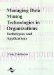Managing Data Mining Technologies in Organizations: Techniques and Applications
ISBN: 1591400570
EAN: 2147483647
Year: 2003
Pages: 174